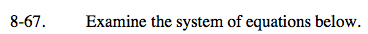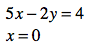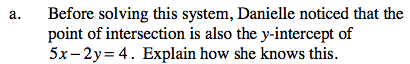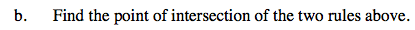### Home > CAAC > Chapter 8 > Lesson 8.2.3 > Problem8-67

8-67.
1.Examine the system of equations below. Homework Help ✎

5x − 2y = 4
x = 0

1. Before solving this system, Danielle noticed that the point of intersection is also the y-intercept of 5x − 2y = 4. Explain how she knows this.

2. Find the point of intersection of the two rules above.The line x = 0 is the y-axis.

The y-intercept is the point at which a line intersects the y-axis.Substitute 0 in for x.

Solve for y.

Intersection = (0, −2)